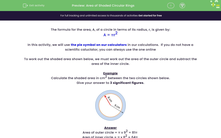# Area of Shaded Circular Rings

In this worksheet, students must calculate the area of shaded circular rings.Key stage:  KS 3

Curriculum topic:   Geometry and Measures

Curriculum subtopic:   Solve Problems Involving Perimeters and Areas of 2D Shapes

Difficulty level:#### Worksheet Overview

The formula for the area, A, of a circle in terms of its radius, r, is given by:

A = πr2

In this activity, we will use the pie symbol on our calculators in our calculations.  If you do not have a scientific caluclator, you can always use the one online

To work out the shaded area shown below, we must work out the area of the outer circle and subtract the area of the inner circle.

Example

Calculate the shaded area in cm2 between the two circles shown below.Area of outer circle = π x 92 = 81π

Area of inner circle = π x 82 = 64π

Shaded area = 81π - 64π = 17π = 53.38 cm2

= 53.4 cm ² (3 s.f.)

### What is EdPlace?

We're your National Curriculum aligned online education content provider helping each child succeed in English, maths and science from year 1 to GCSE. With an EdPlace account you’ll be able to track and measure progress, helping each child achieve their best. We build confidence and attainment by personalising each child’s learning at a level that suits them.

Get started